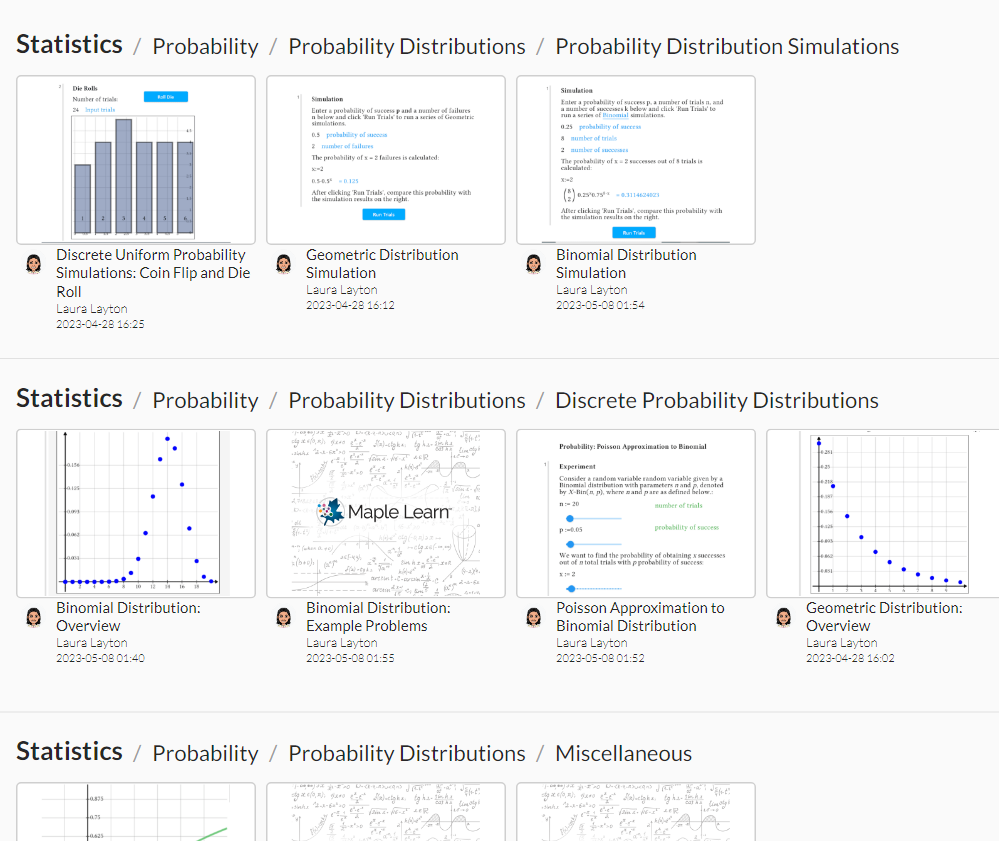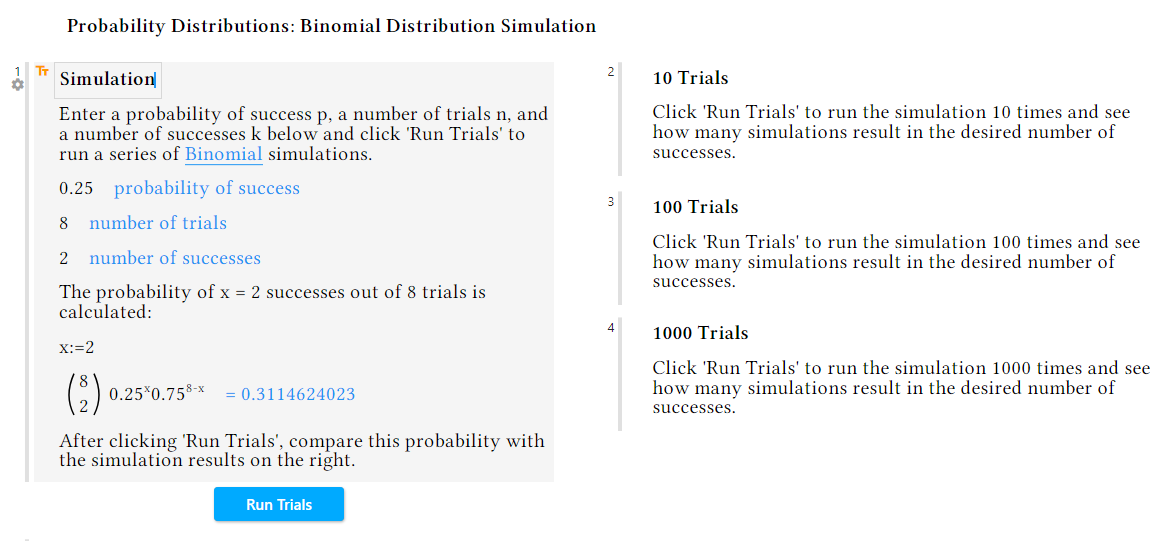## Probability Distributions

Maple Learn

Probability distributions can be used to predict many things in life: how likely you are to wait more than 15 minutes at a bus stop, the probability that a certain number of credit card transactions are fraudulent, how likely it is for your favorite sports team to win at least three games in a row, and many more.

Different situations call for different probability distributions. For instance, probability distributions can be divided into two main categories – those defined by discrete random variables and those defined by continuous random variables. Discrete probability distributions describe random variables that can only take on countable numbers of values, while continuous probability distributions are for random variables that take values from continuums, such as the real number line.

Maple Learn’s Probability Distributions section provides introductions, examples, and simulations for a variety of discrete and continuous probability distributions and how they can be used in real life.One of the distributions highlighted in Maple Learn’s Example Gallery is the binomial distribution. The binomial distribution is a discrete probability distribution that models the number of n Bernoulli trials that will end in a success.

This distribution is used in many real-life scenarios, including the fraudulent credit card transactions scenario mentioned earlier. All the information needed to apply this distribution is the number of trials, n, and the probability of success, p. A common usage of the binomial distribution is to find the probability that, for a recently produced batch of products, the number that are defective crosses a certain threshold; if the probability of having too many defective products is high enough, a company may decide to test each product individually rather than spot-checking, or they may decide to toss the entire batch altogether.

An interesting feature of the binomial distribution is that it can be approximated using a different type of distribution. If the number of trials, n, is large enough and the probability of success, p, is small enough, a Poisson Approximation to the Binomial Distribution can be applied to avoid potentially complex calculations.

To see some binomial distribution calculations in action and how accurate the probabilities supplied by the distribution are, try out the Binomial Distribution Simulation document and see how the Law of Large Numbers relates to your results.You can also try your hand at some Binomial Distribution Example Problems to see some realistic examples and calculations.

Visit the Binomial Distribution: Overview document for a more in-depth explanation of the distribution. The aforementioned Probability Distributions section also contains overviews for the geometric distribution, Poisson distribution, exponential distribution, and several others you may find interesting!﻿